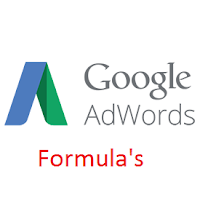source:google

It's important to know how we spend money online in PPC and how to measure the sucess or failure of our Paid Campaign. In this article,I will teach you how to do different calculation in google adword to calculate CTR , CPC , CPA ,ROI ,Clicks , Impression ,Total Budget , Leads , Lead Rate,Ad Rank.

Below there are 10 simple formulas that will help you understand how Adwords costs are calculated and measured:-

Lets Do It

Formula:- Clicks x 100

CTR

Formula:- Impression x CTR
100

100

Formula:-   Clicks  x 100
Impression

Clicks

Formula:- Total Cost
Clicks

Formula:-Clicks x Avg.CPC